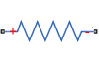## Component Variants — Thermal Resistor

The following example shows a linear resistor with an optional thermal port. The component uses conditional sections to implement the control logic. The `annotations` sections within the conditional branches selectively expose or hide appropriate ports, parameters, and variables based on the value of the control parameter. The two block variants have a different number of ports, and therefore the custom block icon also changes accordingly.

```component CondResistor % Linear Resistor with Optional Thermal Port % If "Model thermal effects" is set to "Off", the block represents a % linear resistor. The voltage-current (V-I) relationship is V=I*R, % where R is the constant resistance in ohms. % % If "Model thermal effects" is set to "On", the block represents a % resistor with a thermal port. The resistance at temperature T1 is given by % R(T) = R0*(1+alpha(T1-T0)), where R0 is the Nominal resistance at the % Reference temperature T0, and alpha is the Temperature coefficient. nodes p = foundation.electrical.electrical; % +:left n = foundation.electrical.electrical; % -:right H = foundation.thermal.thermal; % H:left end parameters thermal_effects = simscape.enum.onoff.off; % Model thermal effects end parameters(ExternalAccess=none) R = { 1, 'Ohm' }; % Nominal resistance T0 = {300,'K'}; % Reference temperature alpha = {50e-6,'1/K'}; % Temperature coefficient tc = {10,'s'}; % Thermal time constant K_d = {1e-3,'W/K'}; % Dissipation factor end variables(ExternalAccess=none) i = { 0, 'A' }; % Current v = { 0, 'V' }; % Voltage T1 = {value = {300,'K'}, priority = priority.high}; % Temperature end branches i : p.i -> n.i; end equations v == p.v - n.v; end if thermal_effects == simscape.enum.onoff.off annotations % Show non-thermal settings Icon = 'custom_resistor.png'; [R, i, v] : ExternalAccess=modify; % Hide thermal node H : ExternalAccess=none; end connections connect(H, *); % Connect hidden thermal node to reference end equations R*i == v; T1 == T0; % Temperature is constant end else annotations % Show thermal settings Icon = 'custom_resistor_thermal.png'; [T1, T0, alpha, tc, K_d, H] : ExternalAccess=modify; end % Add heat flow + thermal equations variables(Access=private) Q = { 0, 'J/s' }; % Heat flow end branches Q : H.Q -> * end equations T1 == H.T; let mc = tc*K_d; % mc in Q = m*c*dT % Calculate R(T), protecting against negative values Rdem = R*(1+alpha*(T1-T0)); R_T = if Rdem > 0, Rdem else {0,'Ohm'} end; in R_T*i == v; % Electrical equation mc * T1.der == Q + R_T*i*i; % Thermal equation end end end end ```

The component initially declares all the optional parameters and variables with the `ExternalAccess` attribute set to `none`, and then exposes them selectively by using the conditional `annotations` sections. The opposite method, of hiding inapplicable members, is also valid, but this approach is more easily scalable when you have multiple component configurations.

If the control parameter, Model thermal effects, is set to `Off`, the block represents a linear resistor. The only exposed block parameter is Nominal resistance, the Initial Targets section lets you set targets for Current and Voltage, and the block icon has two ports, + and -.If the Model thermal effects parameter is set to `On`, the block represents a resistor with a thermal port, with temperature-dependent resistance. The block parameters, variable initialization targets, ports, and the custom block icon change accordingly.